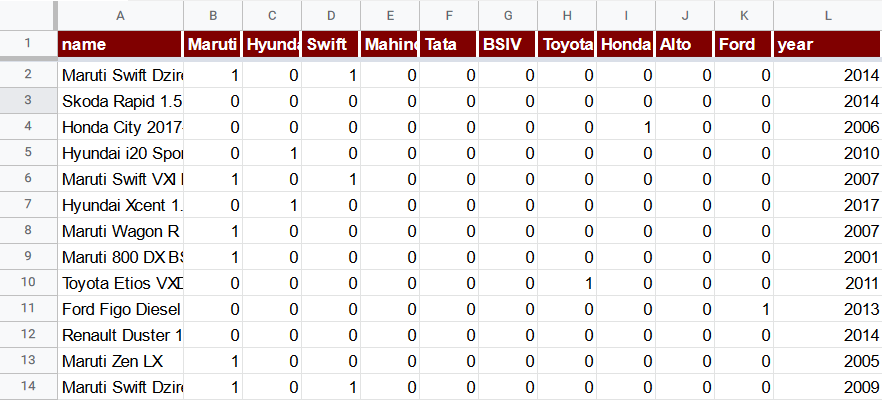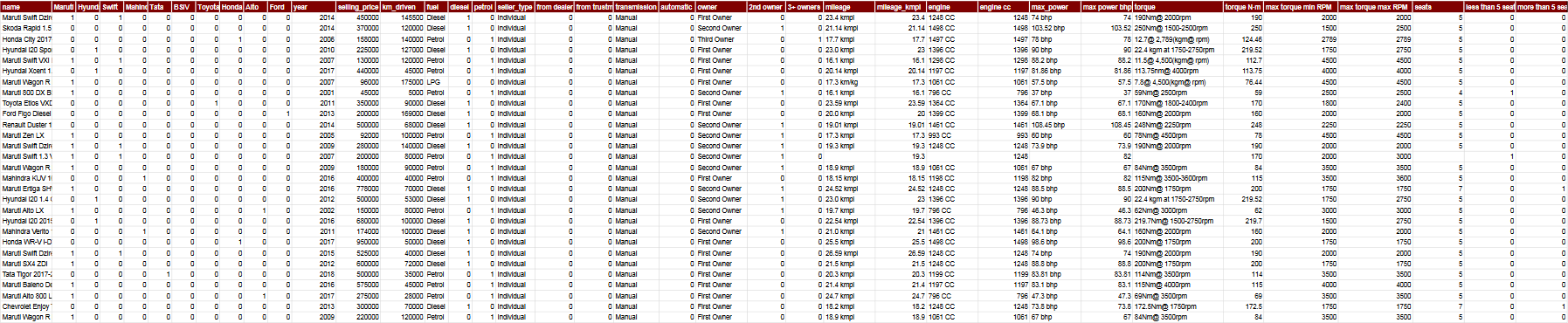## Vehicle dataset

Kaggle provides the vehicle dataset that is very well suited for linear regressions and hence it is used across this documentation for demonstration purposes.

However, non-numeric columns cannot be used in statistical analysis. That’s why we produced a modified version of the dataset with the text values converted into the numeric ones with the appropriate Google Sheets formulas.

If you would like to experiment with the examples provided in TableTorch’s features documentation, it is possible to either:

or

• download the original dataset from Kaggle and refer to the instructions below so as to introduce the very same modifications.

## Preparing the dataset

The archive contains the `Car details v3.csv` file containing all the necessary information. Such columns as year, selling_price, km_driven, seats have a numeric format and do not require any further action. However, the columns of name, fuel, seller_type, transmission, owner, mileage, engine, max_power and torque have a text format and require conversion.

The following sections discuss the conversion of each column, the letter in parentheses is column’s sheet-level identifier. The provided formulas are appropriate for the first data row, Google Sheets usually allows to apply the formula to the rest of the rows automatically by pressing `Ctrl+Enter` after entering the formula for the first row.

### name (A)

`name` is a particularly interesting column because it has no numbers whatsoever and rather identifies the car’s model in a free form. However, as a car model feature may be a significant one in a regression, it would be wasteful not to attempt to extract at least some numeric information from it. One way of doing that is to identify the most frequently occurred words among the names and to add a column with a binary value, i.e. `1` if name contains the word and `0` if it does not.

The top 10 such words for the dataset are Maruti, Hyundai, Swift, Mahindra, Tata, BSIV, Toyota, Honda, Alto, Ford. For each of these words, insert a separate column with an appropriate formula as illustrated below:

#### Maruti (B)

``````=IF(ISNUMBER(FIND("Maruti", A2)), 1, 0)
``````

#### Hyundai (C)

``````=IF(ISNUMBER(FIND("Hyundai", A2)), 1, 0)
``````

#### Swift (D)

``````=IF(ISNUMBER(FIND("Swift", A2)), 1, 0)
``````

#### Mahindra (E)

``````=IF(ISNUMBER(FIND("Mahindra", A2)), 1, 0)
``````

#### Tata (F)

``````=IF(ISNUMBER(FIND("Tata", A2)), 1, 0)
``````

#### BSIV (G)

``````=IF(ISNUMBER(FIND("BSIV", A2)), 1, 0)
``````

#### Toyota (H)

``````=IF(ISNUMBER(FIND("Toyota", A2)), 1, 0)
``````

#### Honda (I)

``````=IF(ISNUMBER(FIND("Honda", A2)), 1, 0)
``````

#### Alto (J)

``````=IF(ISNUMBER(FIND("Alto", A2)), 1, 0)
``````

#### Ford (K)

``````=IF(ISNUMBER(FIND("Ford", A2)), 1, 0)
``````

The sheet now has 10 additional numerically processable columns identifying car manufacturers.### fuel (O)

There are a few different types of fuel present in the dataset, however, the great majority of the records has either of the two: diesel or petrol. Create two columns with the formulas as follows:

#### diesel (P)

``````=IF(O2="Diesel", 1, 0)
``````

#### petrol (Q)

``````=IF(O2="Petrol", 1, 0)
``````

### seller_type (R)

There are three unique types of seller types in the dataset: Individual, Dealer, and Trustmark Dealer. Most of the records are of Individual type hence it makes sense to extract the other two variants into separate columns. Insert two new columns:

### from dealer (S)

``````=IF(R2="Dealer", 1, 0)
``````

### from trustmark dealer (T)

``````=IF(R2="Trustmark Dealer", 1, 0)
``````

### transmission (U)

Transmission is either Manual or Automatic so just a single additional column is enough to extract a numeric value.

### automatic (V)

``````=IF(U2="Automatic", 1, 0)
``````

### owner (W)

Extract two more columns from the owner column for the Second Owner and anything other than First Owner or Second Owner. Rare values are ignored for simplicity.

### 2nd owner (X)

``````=IF(W2="Second Owner", 1, 0)
``````

### 3+ owners (X)

``````=IF(OR(W2="First Owner", W2="Second Owner"), 0, 1)
``````

### mileage (Z)

This is the first column with a concatenated numeric value and the unit of measurement. Furthermore, some records lack any value at all thus it makes sense to supplement them with a median mileage so as to avoid confusing a regression.

The different between kmpl and km/kg units can be ignored for simplicity and a numeric value extracted with the following formula:

### mileage_kmpl (AA)

``````=IF(ISNUMBER(FIND(" ", Z2)), VALUE(LEFT(Z2, FIND(" ", Z2))), 19.3)
``````

### engine (AB)

Insert a conversion column for engine displacement:

### engine cc (AC)

``````=IF(ISNUMBER(FIND(" ", AB2)), VALUE(LEFT(AB2, FIND(" ", AB2))), 1248)
``````

Convert max power to a numeric format with the following formula:

### max power bhp (AE)

``````=IF(ISNUMBER(FIND(" ", AD2)), VALUE(LEFT(AD2, FIND(" ", AD2))), 82)
``````

### torque (AF)

This columns has a harder to parse text format because it features

• two different units of measurement (N·m and kg·m);
• some rows have a range of RPM whereas others only a single value;
• as well as significant variation of the format between the rows.

Insert the following three columns in order to:

• convert all values into a single unit (N·m);
• parse both the minimum and maximum values of the RPM range, using the identical ones if there’s no range in the original value;
• use median values of the appropriate columns where there’s no original information.

### torque N·m (AG)

``````=IF(
ISNUMBER(SEARCH("kgm", AF2)),
VALUE(REGEXEXTRACT(AF2, "[\d\.,]+")) * 9.8,
IF(
ISNUMBER(SEARCH("Nm", AF2)),
VALUE(REGEXEXTRACT(AF2, "[\d\.,]+")),
170
))
``````

### max torque min RPM (AH)

``````=IF(
ISNUMBER(SEARCH("rpm", AF2)),
VALUE(REGEXEXTRACT(AF2, "(?i)[^\d\.,]*[\d\.,]+[^\d\.,]*([\d\.,]+)")),
2000)
``````

### max torque max RPM (AI)

``````=IF(
ISNUMBER(SEARCH("rpm", AF2)),
IF(REGEXMATCH(AF2, "\d-\d"),
VALUE(REGEXEXTRACT(AF2, "(?i)[^\d\.,]*[\d\.,]+[^\d\.,]*[\d\.,]+-([\d\.,]+)")),
VALUE(REGEXEXTRACT(AF2, "(?i)[^\d\.,]*[\d\.,]+[^\d\.,]*([\d\.,]+)"))
),
3000)
``````

### seats (AJ)

Although seats already has a numeric format, it might be useful to extract two additional binary features from it because the value of the car may change non-linearly if the car is not a usual 5-seats car.

### less than 5 seats (AK)

``````=IF(AJ2 < 5, 1, 0)
``````

### more than 5 seats (AL)

``````=IF(AJ2 > 5, 1, 0)
``````

## Conclusion

The table now consists of 38 columns and no longer fits into the screen’s width.Now that almost every possible numeric detail has been extracted from the textual data present in the dataset, it is time to move on to building a model predicting the selling_price column. Review the Correlation matrix and the Linear regression pages to find out how to do that right inside Google Sheets with TableTorch.